# Add Up (Sum) Entire Columns or Rows in Excel

This tutorial demonstrates how to add entire rows or columns in Excel.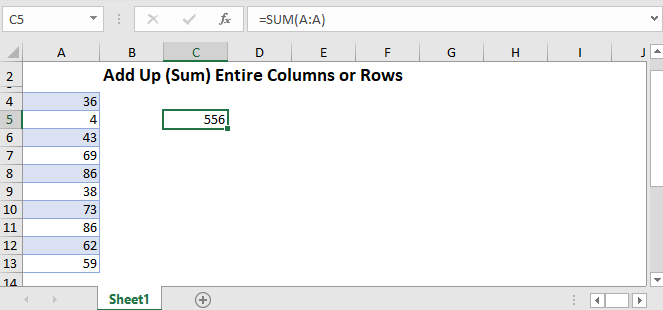## The Sum Function

We will use the Sum Function to add up entire rows and columns. It takes input in two primary forms:

1. Standalone Cell References =sum(a1,b2,c3)
2. Arrays of Cells =sum(A1:E1).

We will use the latter method to sum range A1 to E1:

``=SUM(A1:E1)``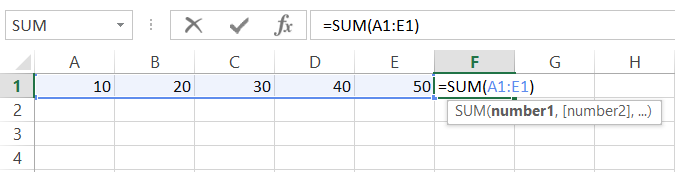Tip 1: The shortcut ALT + = (press and hold ALT then tap =) will automatically create a Sum Function. When possible, Excel will guess which cells you would like to sum together, populating the Sum Function.
Tip 2: After using the ALT + = shortcut or after typing =sum(, use the arrow keys to select the appropriate cell. Then hold down SHIFT or CTRL + SHIFT to select the desired range of cells.
Tip 3: Instead of using the keyboard, you can also use the mouse to drag and highlight the desired range and complete the formula.

## Sum an Entire Column

To add up an entire column, enter the Sum Function: =sum( and then enter the desired column. There are several ways to do this:

• Type the columns “A:A”
• Click the column letter at the top of the worksheet
• Use the arrow keys to navigate to the column and using the CTRL + SPACE shortcut to select the entire column.

The formula will be in the form of.

``=sum(A:A)``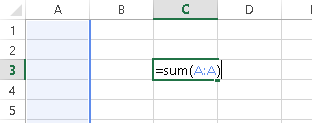## Sum an Entire Row

To sum an entire row, use the same method as with columns:

• Type the rows “3:3”
• Click the row number at the left of the worksheet
• Use the arrow keys to navigate to the column and using the SHIFT + SPACE shortcut to select the entire row.

The formula will be in the form of.

``=sum(1:1)``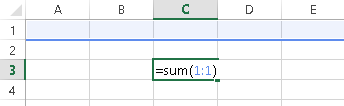## Add up Multiple Columns or Rows at Once

To sum columns or rows at the same time, use a formula of the form: =sum(A:B) or =sum(1:2). Remember that you can also use the keyboard shortcuts CTRL + SPACE to select an entire column or SHIFT + SPACE an entire row. Then, while holding down SHIFT, use the arrow keys to select multiple rows.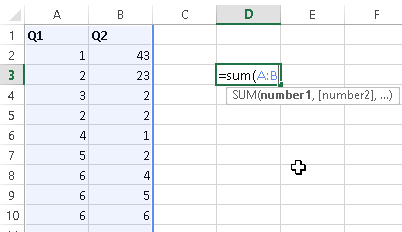## Sum Non-Contiguous Columns or Rows at Once

To sum Non-Contiguous Columns or Rows at Once, enter the separate ranges (columns or rows) separated by commas:

``=SUM(A:A,B:B)``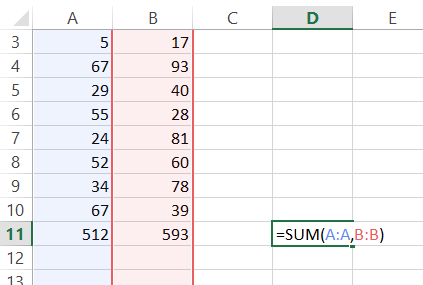## Sum Everything Except the Header

You may need to add up an entire column (or row), except the header. Non-numerical values will be automatically excluded, but if the header is numeric, the pervious methods will not work well

Instead, you can use a formula like this

``=SUM(A2:A1048576)``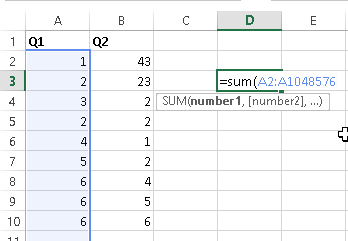Why 1,048,576? Excel worksheets only have 1,048,576 rows!

To see how many rows Excel has, select a cell in a blank column then you use the shortcut: CTRL + Down Arrow to navigate to the last row in the worksheet.

### Easy Excel Automation## Sum to End of Column

Instead of adding up an entire column to the bottom of the worksheet, you can add-up only the rows containing data. To do this, first start your SUM Function. Then select the first row in the column containing the data you wish to sum, then use CTRL + SHIFT + Down Arrow to select all the cells in that column (Note: be careful of blank cells. CTRL + SHIFT + Arrow will navigate to the cell directly before a blank cell)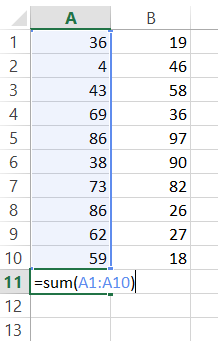## AutoSum Magic

Select a cell above/below or left/right the range you want to sum. Then use the shortcut ALT + = or select the Formulas Ribbon > AutoSum. Doing so will automatically generate a formula for you!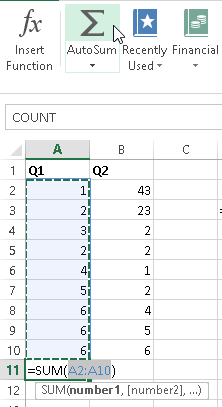## Common Sum Errors

#VALUE! – you have non-integers in the sum formula (not an issue in Excel 2013+)

#REF! – previously referenced column(s) or row(s) does not exist anymore

#NAME? – check formula spelling

## Sum Entire Rows or Columns in Google Sheets

All the examples work the same in Google Sheets as Excel, except (annoyingly) you can not use the keyboard shortcuts to select entire rows or columns.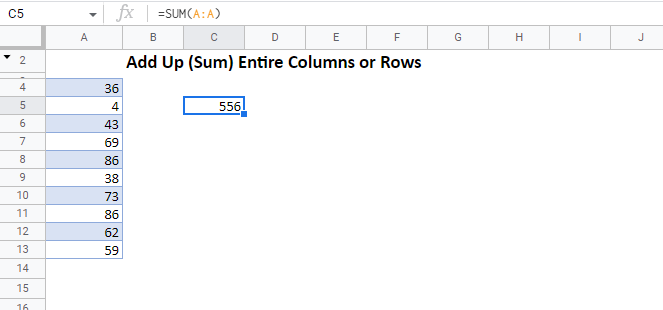### Excel Practice Worksheet

Practice Excel functions and formulas with our 100% free practice worksheets!